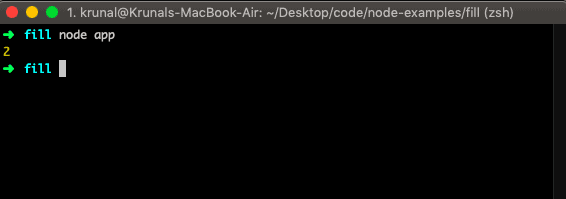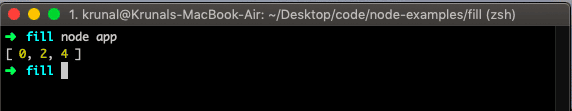# JavaScript array indexOf: The Complete Guide

To find a specified value in the string, use the Javascript String indexOf() method. If you find an index of a particular item in the Array, use the Javascript array indexOf() method.

## JavaScript array indexOf

The array indexOf() is a built-in JavaScript function that returns the first index at which the given item can be found in an array, or -1 if it is not present. Thus, the array indexOf() method searches the Array for a specified item and returns its position to the calling function.

The search will start at the given position, begin if no start position is given, and end the search at the end of an array.

Javascript array indexOf() method returns the first occurrence of a specified value in a string or an array. The indexOf() method returns -1 if the value to search for never occurs. Note: The indexOf() method is case-sensitive.

If you are a JavaScript developer, you are probably familiar with the string indexOf() method on strings.

The primary functionality of Array’s indexOf is very similar to the string indexOf() method.

If the array item is present more than once, the indexOf method returns the position of the first occurrence.

The first element has position 0; the second element has position 1, and so on.

### Syntax

`array.indexOf(item, start)`

### Parameters

The item parameter is required, and its element, which we are searching inside an array.

The start parameter is optional. It is the position where to start the search.

### Return Value

The array indexOf() function returns the integer, the index of an element in the Array.

### Example

Let us take a simple example by creating a file called the app.js and adding the following code.

```// app.js

let pieces = ['king', 'queen', 'rook', 'knight', 'bishop'];

console.log(pieces.indexOf('rook'));```The indexOf() compares searchElement to elements of the Array using strict equality (the same method used by the === or triple-equals operator).

## Finding all the occurrences of an element

Finding all the occurrences of an element in a specific array is a common task we face in web development. Let us take a use case where we need to find all the events of the particular item.

```// app.js

let indices = [];
let array = ['a', 'b', 'a', 'c', 'a', 'd'];
let element = 'a';
let ids = array.indexOf(element);
while (ids != -1) {
indices.push(ids);
ids = array.indexOf(element, ids + 1);
}
console.log(indices);```

In the above example, we search for one item until the whole Array is not searched. Then, if the item’s position is found, it will be inside an array and, finally, log that Array.## Difference between for loop and indexOf()

Suppose that you have an array representing an ordered rank of elements, and you need to find out just where a given element falls in the ranking. Without indexOf, we would need to do something like this.

```// app.js

const data = [
'Millie Bobby Brown',
'Winona Ryder'
];

for (var i = 0;
i < data.length && data[i] !== "Sadie Sink";
i++) {
}

let rank = i + 1;

console.log("Sadie Sink's rank is: " + rank);
```

See the output.

```➜  es git:(master) ✗ node app
➜  es git:(master) ✗
```

Now, with the help of the JavaScript indexOf() method, we no longer have to keep track of the state of our counters and array elements.

Let’s see the above example using the JavaScript indexOf() method.

```// app.js

const data = [
'Millie Bobby Brown',
'Winona Ryder'
];

let rank = index + 1;

console.log("Sadie Sink's rank is: " + rank);
```

It gives us the same output.

## Javascript Array indexof object

To get an index of an array that contains objects, use the Javascript map() method. See the following code example in which we will find the index of a mike, which is 0 basically because when we add the two objects in the Array, then st is the first object, meaning its index is 0.

```// app.js

let st = {
mike: 'Finn',
el: 'Millie'
};
let got = {
jon: 'Kit',
khaleesi: 'Emilia'
}

let arr = [];
arr.push(st, got);
pos = arr.map(function(e) { return e.mike; }).indexOf('Finn');

console.log(pos);
```

In the above example, first, we have two objects.

1. st
2. got

We define one Array called arr and add the two objects using the Javascript push() method.

Now, we need to find the index of the mike key, whose value is Finn.

But, the object is st, and in the Array, it is in the first position, and the array index starts with 0. So the index of mike is also 0. So, in the output, we will get the 0 as the mike key and Finn value position. See the output.

```➜  es git:(master) ✗ node app
0
➜  es git:(master) ✗
```

## The array indexof() method is case insensitive.

To search an element in the array regardless of its case sensitiveness, use the Javascript array findIndex() and Javascript toLowerCase() method.

See the following code example.

```// app.js

let arr = ['miLLie', 'fiNN', 'saDiE', 'caLEB', 'gaTEn'];
query = 'caleb',
pos = arr.findIndex(item => query.toLowerCase() === item.toLowerCase());
console.log(result);
```

In the above example, we have defined one array item, and we need to search one array item, defined as a query.

Regardless of case sensitiveness, we need to find the item from that Array.

So, we have used the Array findIndex() method to get the exact position of the element and return that position. See the output.

```➜  es git:(master) ✗ node app
3
➜  es git:(master) ✗
```

We are searching for the caleb element, which has an index of 3.

## Browser and Node Compatibility(Version and above)

2. Internet Explorer v3
3. Firefox v1
4. Edge v12
5. Opera
6. Safari v1
7. Android webview v1
8. Chrome for Android v18
9. Firefox for Android v4
10. Opera for Android
11. Safari on iOS v1
12. Samsung Internet v1.0
13. Node.js

That’s it for this tutorial.

## Recommended Posts

Javascript array find()

Javascript array lastIndexOf()

Javascript array filter()

Javascript array forEach()

Javascript array toString

### 1 thought on “JavaScript array indexOf: The Complete Guide”

1.thanks for the guide